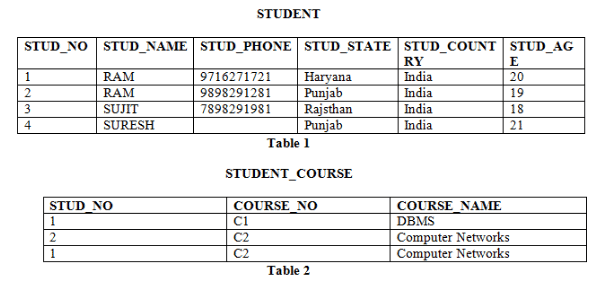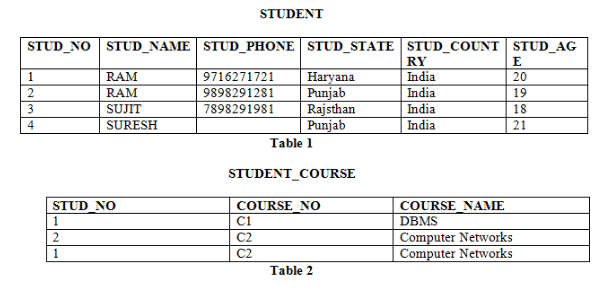Courses

# Relational Model Computer Science Engineering (CSE) Notes | EduRev

## Mock Test Series - Computer Science Engg. (CSE) GATE 2020

Created by: Gate Gurus

## Computer Science Engineering (CSE) : Relational Model Computer Science Engineering (CSE) Notes | EduRev

The document Relational Model Computer Science Engineering (CSE) Notes | EduRev is a part of the Computer Science Engineering (CSE) Course Mock Test Series - Computer Science Engg. (CSE) GATE 2020.
All you need of Computer Science Engineering (CSE) at this link: Computer Science Engineering (CSE)

Relational Model

Relational Model was proposed by E.F. Codd to model data in the form of relations or tables. After designing the conceptual model of Database using ER diagram, we need to convert the conceptual model in the relational model which can be implemented using any RDMBS languages like Oracle SQL, MySQL etc. So we will see what Relational Model is.

What is Relational Model?

Relational Model represents how data is stored in Relational Databases.  A relational database stores data in the form of relations (tables). Consider a relation STUDENT with attributes ROLL_NO, NAME, ADDRESS, PHONE and AGE shown in Table 1.

STUDENT

 ROLL_NO NAME ADDRESS PHONE AGE 1 RAM DELHI 9455123451 18 2 RAMESH GURGAON 9652431543 18 3 SUJIT ROHTAK 9156253131 20 4 SURESH DELHI 18

IMPORTANT TERMINOLOGIES

1. Attribute: Attributes are the properties that define a relation. e.g.; ROLL_NONAME
2. Relation Schema: A relation schema represents name of the relation with its attributes. e.g.; STUDENT (ROLL_NO, NAME, ADDRESS, PHONE and AGE) is relation schema for STUDENT. If a schema has more than 1 relation, it is called Relational Schema.
3. Tuple: Each row in the relation is known as tuple. The above relation contains 4 tuples, one of which is shown as:

 1 RAM DELHI 9455123451 18

1. Relation Instance: The set of tuples of a relation at a particular instance of time is called as relation instance. Table 1 shows the relation instance of STUDENT at a particular time. It can change whenever there is insertion, deletion or updation in the database.
2. Degree: The number of attributes in the relation is known as degree of the relation. The STUDENT relation defined above has degree 5.
3. CardinalityThe number of tuples in a relation is known as cardinality. The STUDENT relation defined above has cardinality 4.
4. Column: Column represents the set of values for a particular attribute. The column ROLL_NO is extracted from relation STUDENT.

 ROLL_NO 1 2 3 4

1. NULL Values: The value which is not known or unavailable is called NULL value. It is represented by blank space. e.g.; PHONE of STUDENT having ROLL_NO 4 is NULL.

Constraints in Relational Model

While designing Relational Model, we define some conditions which must hold for data present in database are called Constraints. These constraints are checked before performing any operation (insertion, deletion and updation) in database. If there is a violation in any of constrains, operation will fail.

Domain Constraints: These are attribute level constraints. An attribute can only take values which lie inside the domain range. e.g,; If a constrains AGE>0 is applied on STUDENT relation, inserting negative value of AGE will result in failure.

Key Integrity: Every relation in the database should have atleast one set of attributes which defines a tuple uniquely. Those set of attributes is called key. e.g.; ROLL_NO in STUDENT is a key. No two students can have same roll number. So a key has two properties:

• It should be unique for all tuples.
• It can’t have NULL values.

Referential Integrity: When one attribute of a relation can only take values from other attribute of same relation or any other relation, it is called referential integrity. Let us suppose we have 2 relations

STUDENT

 ROLL_NO NAME ADDRESS PHONE AGE BRANCH_CODE 1 RAM DELHI 9455123451 18 CS 2 RAMESH GURGAON 9652431543 18 CS 3 SUJIT ROHTAK 9156253131 20 ECE 4 SURESH DELHI 18 IT

BRANCH

 BRANCH_CODE BRANCH_NAME CS COMPUTER SCIENCE IT INFORMATION TECHNOLOGY ECE ELECTRONICS AND COMMUNICATION ENGINEERING CV CIVIL ENGINEERING

BRANCH_CODE of STUDENT can only take the values which are present in BRANCH_CODE of BRANCH which is called referential integrity constraint. The relation which is referencing to other relation is called REFERENCING RELATION (STUDENT in this case) and the relation to which other relations refer is called REFERENCED RELATION (BRANCH in this case).

ANOMALIES

An anomaly is an irregularity, or something which deviates from the expected or normal state. When designing databases, we identify three types of anomalies: Insert, Update and Delete.

Insertion Anomaly in Referencing Relation:

We can’t insert a row in REFERENCING RELATION if referencing attribute’s value is not present in referenced attribute value. e.g.; Insertion of a student with BRANCH_CODE ‘ME’ in STUDENT relation will result in error because ‘ME’ is not present in BRANCH_CODE of BRANCH.

Deletion/ Updation Anomaly in Referenced Relation:

We can’t delete or update a row from REFERENCED RELATION if value of REFRENCED ATTRIBUTE is used in value of REFERENCING ATTRIBUTE. e.g; if we try to delete tuple from BRANCH having BRANCH_CODE ‘CS’, it will result in error because ‘CS’ is referenced by BRANCH_CODE of STUDENT, but if we try to delete the row from BRANCH with BRANCH_CODE CV, it will be deleted as the value is not been used by referencing relation. It can be handled by following method:

ON DELETE CASCADE: It will delete the tuples from REFERENCING RELATION if  value used by REFERENCING ATTRIBUTE is deleted from REFERENCED RELATION. e.g;, if we delete a row from BRANCH with BRANCH_CODE ‘CS’, the rows in STUDENT relation with BRANCH_CODE CS (ROLL_NO 1 and 2 in this case) will be deleted.

ON UPDATE CASCADE: It will update the REFERENCING ATTRIBUTE in REFERENCING RELATION if attribute value used by REFERENCING ATTRIBUTE is updated in REFERENCED RELATION. e.g;, if we update a row from BRANCH with BRANCH_CODE ‘CS’ to ‘CSE’, the rows in STUDENT relation with BRANCH_CODE CS (ROLL_NO 1 and 2 in this case) will be updated with BRANCH_CODE ‘CSE’.

DBMS | Relational Model Introduction and Codd Rules

Terminology

Relational Model: Relational model represents data in the form of relations or tables.

Relational Schema: Schema represents structure of a relation. e.g.; Relational Schema of STUDENT relation can be represented as:
STUDENT (STUD_NO, STUD_NAME, STUD_PHONE, STUD_STATE, STUD_COUNTRY, STUD_AGE)

Relational Instance: The set of values present in a relation at a particular instance of time is known as relational instance as shown in Table 1 and Table 2.

Attribute: Each relation is defined in terms of some properties, each of which is known as attribute. For Example, STUD_NO, STUD_NAME etc. are attributes of relation STUDENT.

Domain of an attribute: The possible values an attribute can take in a relation is called its domain. For Example, domain of STUD_AGE can be from 18 to 40.
Tuple: Each row of a relation is known as tuple. e.g.; STUDENT relation given above has 4 tuples.

NULL values: Values of some attribute for some tuples may be unknown, missing or undefined which are represented by NULL. Two NULL values in a relation are considered different from each other.
Table 1 and Table 2 represent relational model having two relations STUDENT and STUDENT_COURSECodd Rules

Codd rules were proposed by E.F. Codd which should be satisfied by relational model.

1. Information Rule: Data stored in Relational model must be a value of some cell of a table.
2. Guaranteed Access Rule: Every data element must be accessible by table name, its primary key and name of attribute whose value is to be determined.
3. Systematic Treatment of NULL values: NULL value in database must only correspond to missing, unknown or not applicable values.
4. Active Online Catalog: Structure of database must be stored in an online catalog which can be queried by authorized users.
5. Comprehensive Data Sub-language Rule: A database should be accessible by a language supported for definition, manipulation and transaction management operation.
6. View Updating Rule: Different views created for various purposes should be automatically updatable by the system.
7. High level insert, update and delete rule: Relational Model should support insert, delete, update etc. operations at each level of relations. Also, set operations like Union, Intersection and minus should be supported.
8. Physical data independence: Any modification in the physical location of a table should not enforce modification at application level.
9. Logical data independence: Any modification in logical or conceptual schema of a table should not enforce modification at application level. For example, merging of two tables into one should not affect application accessing it which is difficult to achieve.
10. Integrity Independence: Integrity constraints modified at database level should not enforce modification at application level.
11. Distribution Independence: Distribution of data over various locations should not be visible to end-users.
12. Non-Subversion Rule: Low level access to data should not be able to bypass integrity rule to change data.

Given the basic ER and relational models, which of the following is INCORRECT?

A. An attribute of an entity can have more than one value
B. An attribute of an entity can be composite
C. In a row of a relational table, an attribute can have more than one value
D. In a row of a relational table, an attribute can have exactly one value or a NULL value

Answer: In relation model, an attribute can’t have more than one value. So, option C is the answer.

DBMS | Keys in Relational Model (Candidate, Super, Primary, Alternate and Foreign)

We strongly recommend to refer below post as a prerequisite of this.

DBMS | Relational Model introduction and code Rules

Different Types of Keys in Relational ModeCandidate Key: The minimal set of attribute which can uniquely identify a tuple is known as candidate key. For Example, STUD_NO in STUDENT relation.

• The value of Candidate Key is unique and non-null for every tuple.
• There can be more than one candidate key in a relation. For Example, STUD_NO as well as STUD_PHONE both are candidate keys for relation STUDENT.
• The candidate key can be simple (having only one attribute) or composite as well. For Example, {STUD_NO, COURSE_NO} is a composite candidate key for relation STUDENT_COURSE.

Super Key: The set of attributes which can uniquely identify a tuple is known as Super Key. For Example, STUD_NO, (STUD_NO, STUD_NAME) etc.

• Adding zero or more attributes to candidate key generates super key.
• A candidate key is a super key but vice versa is not true.

Primary Key: There can be more than one candidate key in a relation out of which one can be chosen as primary key. For Example, STUD_NO as well as STUD_PHONE both are candidate keys for relation STUDENT but STUD_NO can be chosen as primary key (only one out of many candidate keys).

Alternate Key: The candidate key other than primary key is called as alternate key. For Example, STUD_NO as well as STUD_PHONE both are candidate keys for relation STUDENT but STUD_PHONE will be alternate key (only one out of many candidate keys).

Foreign Key: If an attribute can only take the values which are present as values of some other attribute, it will be foreign key to the attribute to which it refers. The relation which is being referenced is called referenced relation and corresponding attribute is called referenced attribute and the relation which refers to referenced relation is called referencing relation and corresponding attribute is called referencing attribute. Referenced attribute of referencing attribute should be primary key. For Example, STUD_NO in STUDENT_COURSE is a foreign key to STUD_NO in STUDENT relation.

Offer running on EduRev: Apply code STAYHOME200 to get INR 200 off on our premium plan EduRev Infinity!

,

,

,

,

,

,

,

,

,

,

,

,

,

,

,

,

,

,

,

,

,

;